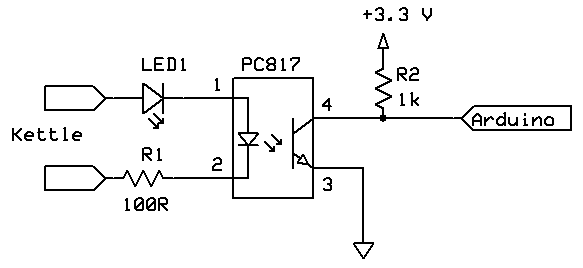# Which resistors fpr PC817 relay?

Hi!
I want to use an PC817 SSD relay to drop 24V against zero potential (odometer counter).
Which resistor should I use to make sure, the PC817 isn't damaged?

Thanks!For 24 Vols ("kettle") side you need a 1.2K 0.5Watt resistor (R1).
R2 can be 1K-5K

I don't know what kind of Arduino you have, so 3.3V could be a 5V

to drop 24V against zero potential (odometer counter).

That's not enough information. Which "side" is the odometer/counter on? Where are the specs to the odometer/counter?

What's on the other side? An Arduino input? An Arduino output? Something else?

I want to use an PC817 SSD relay

That's an optical isolator or opto-coupler, not a solid state relay. (The differences can be subtle.)

To properly use an opto coupler you have to understand CTR (Current Transfer Ratio).
For an average PC817 (there are several grades) I think it's 50% (worst case).
That means opto LED current has to be at least twice the transistor current.
Since the transistor current can be 3.3volt/1k = 3.3mA, you need at least 6.6mA LED current.
An opto LED (IR LED) has a Vf of about 1.2volt, meaning there is about 22.8volt across the resistor
(assuming 24volt input).
The resistor then works out to 22.8volt/0.0066A = 3454ohm. A 3k3 resistor will do.
Could go as low as 1k, for 22.8mA LED current, but all it does is burn more power in the resistor.
2k2 (~10mA LED current) seems a good average.
Leo..

Thanks for the replies!
Ok. I think my explanation wasn't goot enough. The arduino should control the odometer (which is in fact an odomotet "input" of a measurement device), not should the odometer impulse be measured by arduino.

I think I should have mentioned that (sorry).

So. The PC817 should "connect" my 24V (input) pin to the ground (input) pin. My arduino applies 5V to the PC817.

For that case I need the save resistor values.The 1k resistor is far too low and indeed, unnecessary. Given that the opto-coupler is adjacent to the Arduino, you just use INPUT_PULLUP on that pin. If you must use a pull-up, 10k or 22k. Then you need far less current to the input of the opto and far less dissipation in the input resistor.

According

Paul__B:
The 1k resistor is far too low and indeed, unnecessary. Given that the opto-coupler is adjacent to the Arduino, you just use INPUT_PULLUP on that pin. If you must use a pull-up, 10k or 22k. Then you need far less current to the input of the opto and far less dissipation in the input resistor.

According to previous post (#6)

CPIIVU:
The arduino should control the odometer (which is in fact an odomotet "input" of a measurement device), not should the odometer impulse be measured by arduino.
So. The PC817 should "connect" my 24V (input) pin to the ground (input) pin. My arduino applies 5V to the PC817.

So the right side of shown schematic should be connected not to arduino but to odometer. And it's not clear if odometer input has a pull-up. So 5K-20K pull up is necessary. And I'd put 22R resistor between transistor collector and an odometer input.

Well, to characterise an unknown device, you need to have it powered and then connect your multimeter on the milliamps range, firstly between the input and ground, and then between the input and the relevant supply voltage.

If you are not sure what the relevant supply voltage is, then first use the multimeter on Volts between the input and ground to see if there is any pull-up at all inside and then on Volts, between the input and the initial supply voltage of the device such as 12 V. If there is something like a CMOS logic level device inside, this will indicate what its input clamping diodes hold it to compared to the initial supply voltage to an internal regulator.

If you do not follow this, please explain properly what device you propose to use on the input of the PC817 and what this odometer is; at what voltage it operates - is this 24 V - and what its original application is; to what is it normally connected?